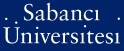# On the Carlitz rank of permutation polynomials

Aksoy, Esen and Çeşmelioğlu, Ayça and Meidl, Wilfried and Topuzoğlu, Alev (2009) On the Carlitz rank of permutation polynomials. Finite Fields and Their Applications, 15 (4). pp. 428-440. ISSN 1071-5797

This is the latest version of this item.

Full text not available from this repository.

Official URL: http://dx.doi.org/10.1016/j.ffa.2009.02.006

## Abstract

A well-known result of Carlitz, that any permutation polynomial p(x) of a finite field F-q is a composition of linear polynomials and the monomial x(q-2). implies that V(x) can be represented by a polynomial P-n(x) = (...((a(0)x + a(1))(q-2) + a(2))(q-2)...+ a(n))(q-2) + a(n+1). for some n >= 0. The smallest integer n, such that P,,(x) represents p(x) is of interest since it is the least number of "inversions" x(q-2), needed to obtain p(x). We define the Carlitzrank of p(x) as n, and focus here on the problem of evaluating it. We also obtain results on the enumeration of permutations of F-q with a fixed Carlitz rank.

Item Type: Article Permutation polynomials of finite fields; Carlitz rank; Enumeration of permutation polynomials; Stirling numbers of the first kind Q Science > QA Mathematics > QA150-272.5 Algebra 13230 Alev Topuzoğlu 03 Dec 2009 16:48 25 May 2011 14:20

### Available Versions of this Item

Repository Staff Only: item control page